Building a Visualization of Solution Behavior

Assuming that β and γ are real, the solutions can be characterized by the roots of the quadratic equation. That is, our solution is known to be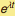:  the solution monotonically grows or shrinks in time if the roots are real-positive or real-negative. The condition that the roots are real is γ <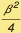If the roots have an imaginary part, the solutions can oscillate. Whether the oscillation amplitudes grow or shrink depends on whether the real part of λ is positive or negative. We will calculate conditions that will indicate the nature of solutions:  if the solution is complex, the imaginary part will determine the frequency of the oscillatory solutions. In all cases, the sign of the real part will indicate whether the amplitude will grow exponentially (and become unbounded) or shrink asymptotically towards zero.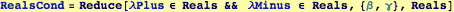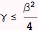The Complex and Real Domains

We use RealsCond and RegionPlot to indicate domain where solutions are real or complex.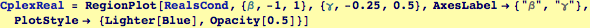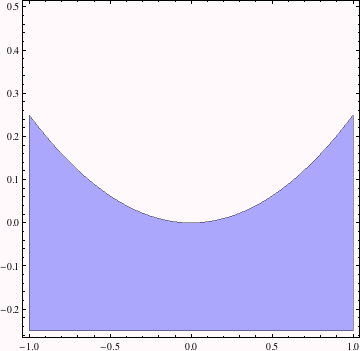Annotate the regions and update the assignment to a new combined graphic.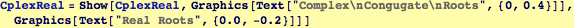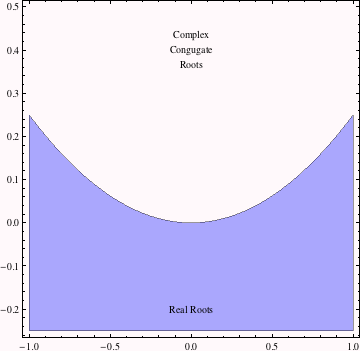The Sign of the Real Part of Complex Solutions

If the roots complex, then the sign of the real part is determined only by the sign of β; i.e., λ =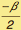±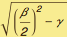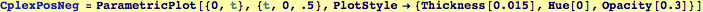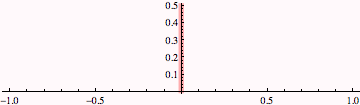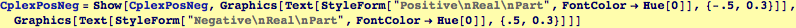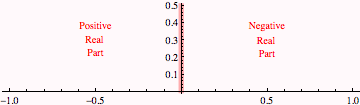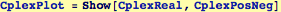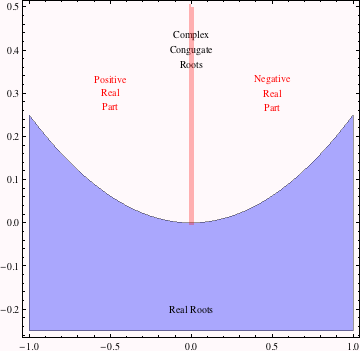Real Solutions, Both Positive or Both Negative

Find the conditions where the real roots are positive and/or negative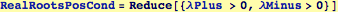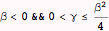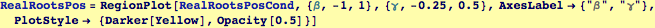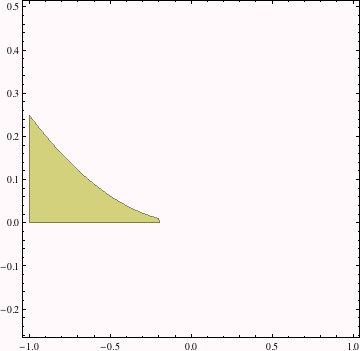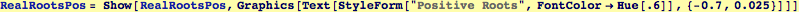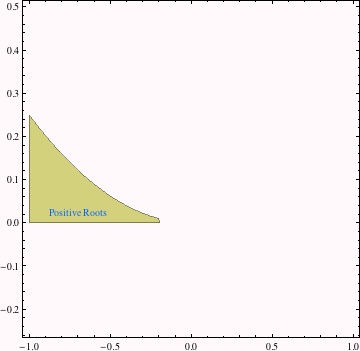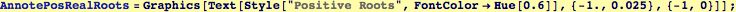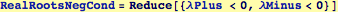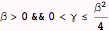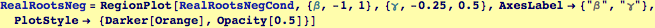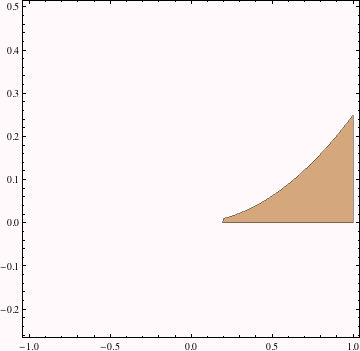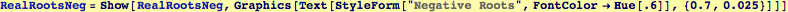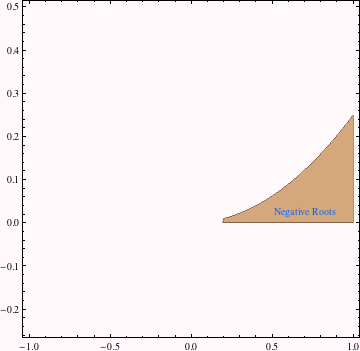Real Solutions, Opposite Signs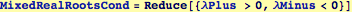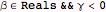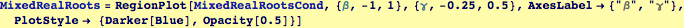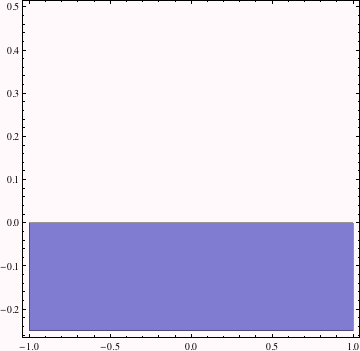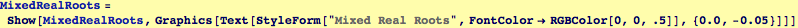Put it all together for a complete graphic....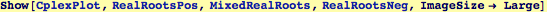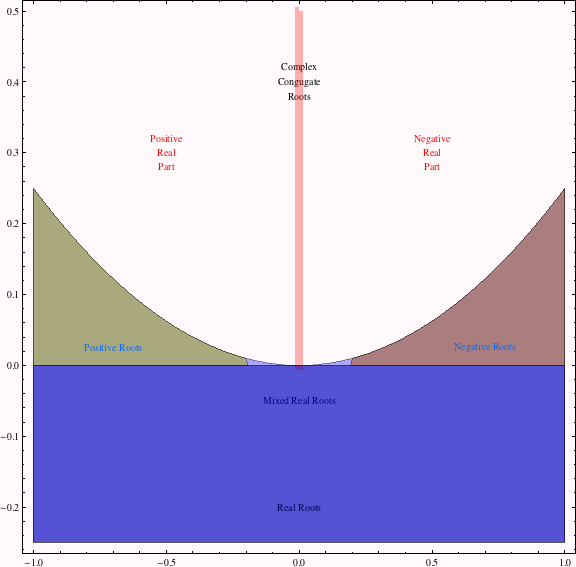Created by Wolfram Mathematica 6.0  (16 November 2007)